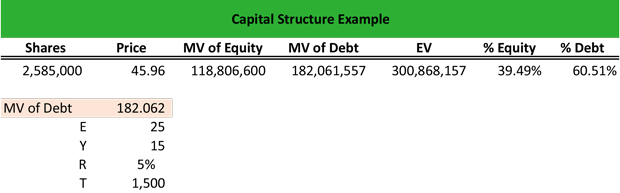# What is Capital Structure?

Definition: Capital structure is the allocation of debt and equity that a firm uses to fund its operations and expansions.

## What Does Capital Structure Mean?

What is the definition of capital structure? Equity capital is the funds that the firm’s owners are trusting with the company and the retained earnings that represent profits from previous years, which are not distributed to the shareholders as dividends but are used towards the financing of debt or expansion of business.

Debt capital represents the borrowed funds of a firm, usually long-term bonds to mitigate interest rate risk over a longer maturity. Firms seek to optimize their capitalization structure to achieve financial flexibility and stronger financial results. Optimizing means to achieve a debt-to-equity ratio that is lower or in line with the industry average. To calculate the capitalization structure, we need to know the market value of equity and the market value of debt.

Let’s look at an example.

## Example

Company A is an equity investment firm that undertakes various clients, among them firms that operate in the electricity sector. Maria wants to calculate the capitalization structure for an electricity company. The electricity company has 2,585,000 shares outstanding, which trade at \$45.96, hence its market cap (market equity) is \$118,806,600 billion.

Maria calculates the market value of debt as follows:

The cost of debt is 5% for 15-year maturity bonds at an annual interest expense of \$25 million and a total debt amount of \$1.5 billion. Therefore:

The market value of debt = E ((1-(1/(1+R) ^Y)) /R) + T/(1 + R) ^Y = \$25 ((1-(1/1+0.05) ^15)) /0.05 + 1,500/(1+0.05) ^15 = \$182,061,557 million.

Hence, the enterprise value of the company is the market value of equity + market value of debt = \$118,806,600 + \$182,061,557 = \$300,868,157.Therefore, Maria calculates that the percentage of equity is \$118,806,800 / \$300,868,157 = 39.49%. Consequently, the percentage of debt is 60.51%.

To calculate the debt to equity ratio of the electricity firm, Maria divides the firm’s liabilities by the equity.

## Summary Definition

Define Capital Structure: Cap structure means the mix of equity and debt financing a company uses to fund its business operations.